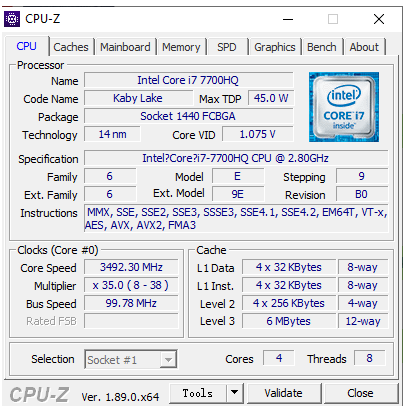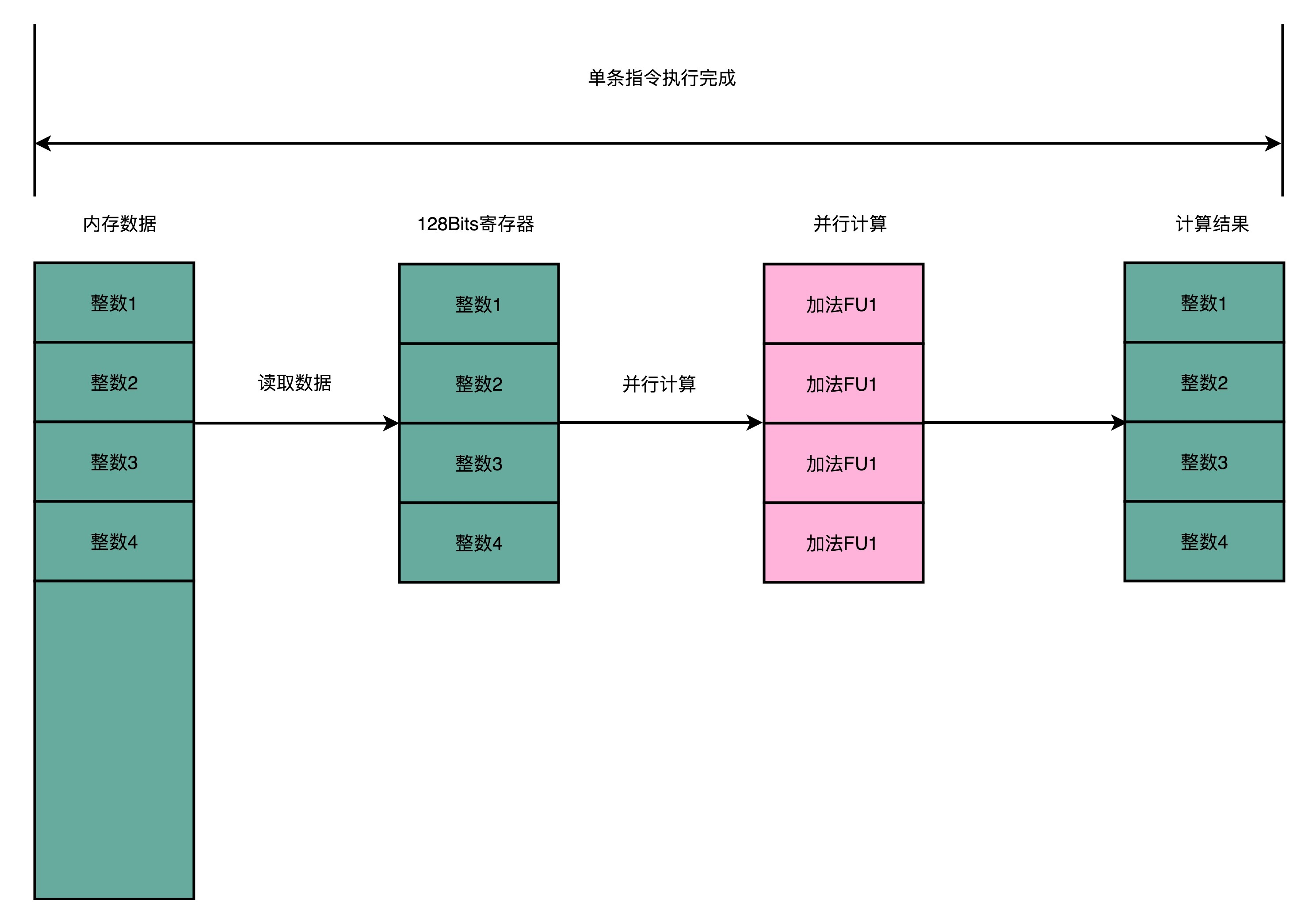27 SIMD：如何加速矩阵乘法？

超线程：Intel 多卖给你的那一倍 CPUSIMD：如何加速矩阵乘法？

\$ python
>>> import numpy as np
>>> import timeit
>>> a = list(range(1000))
>>> b = np.array(range(1000))
>>> timeit.timeit("[i + 1 for i in a]", setup="from __main__ import a", number=1000000)
32.82800309999993
>>> timeit.timeit("np.add(1, b)", setup="from __main__ import np, b", number=1000000)
0.9787889999997788
>>>